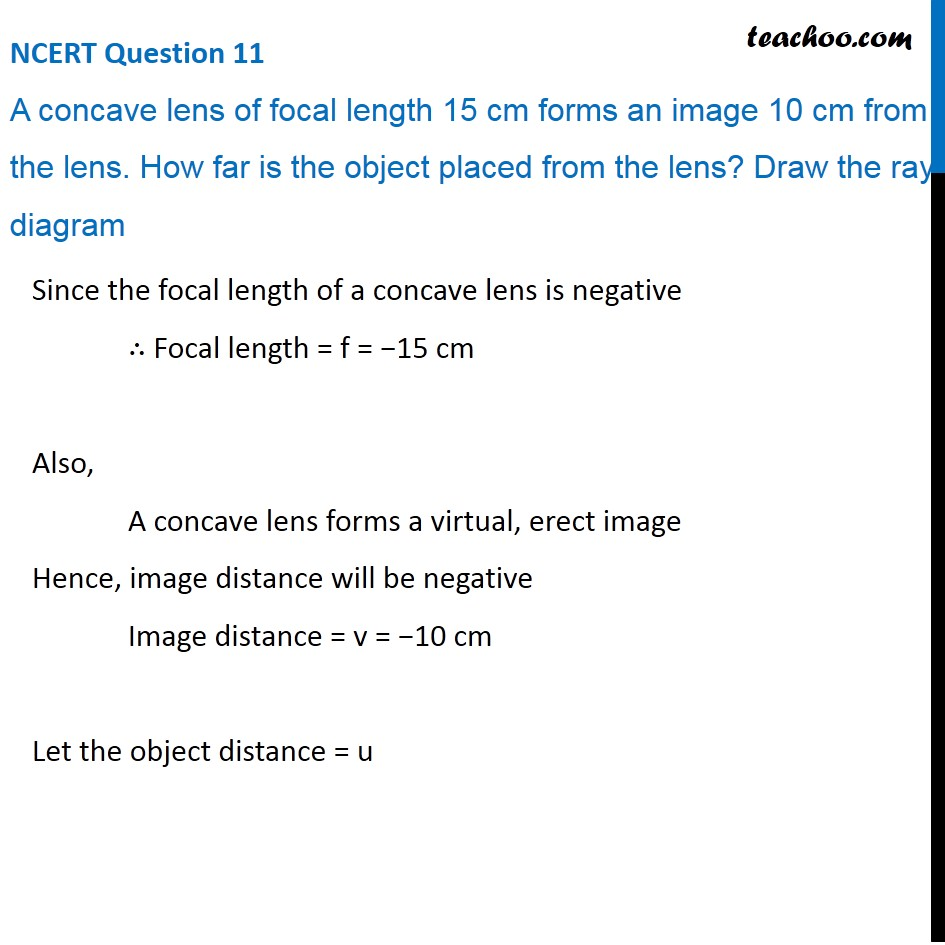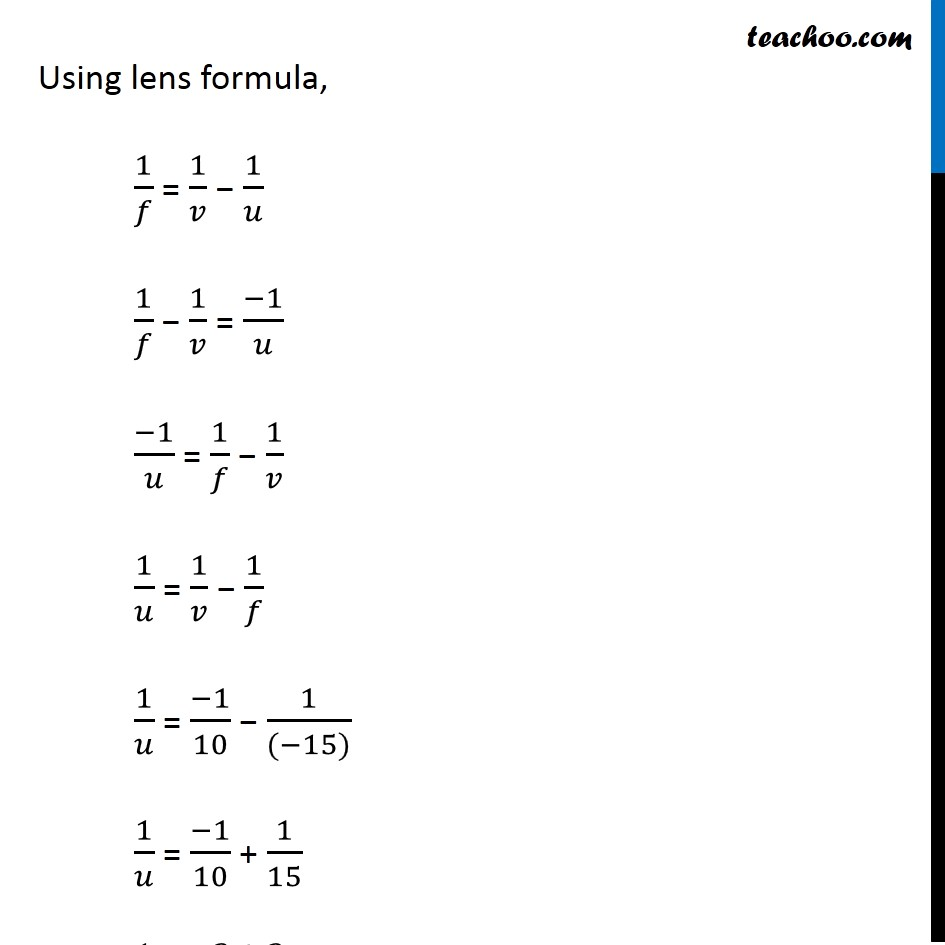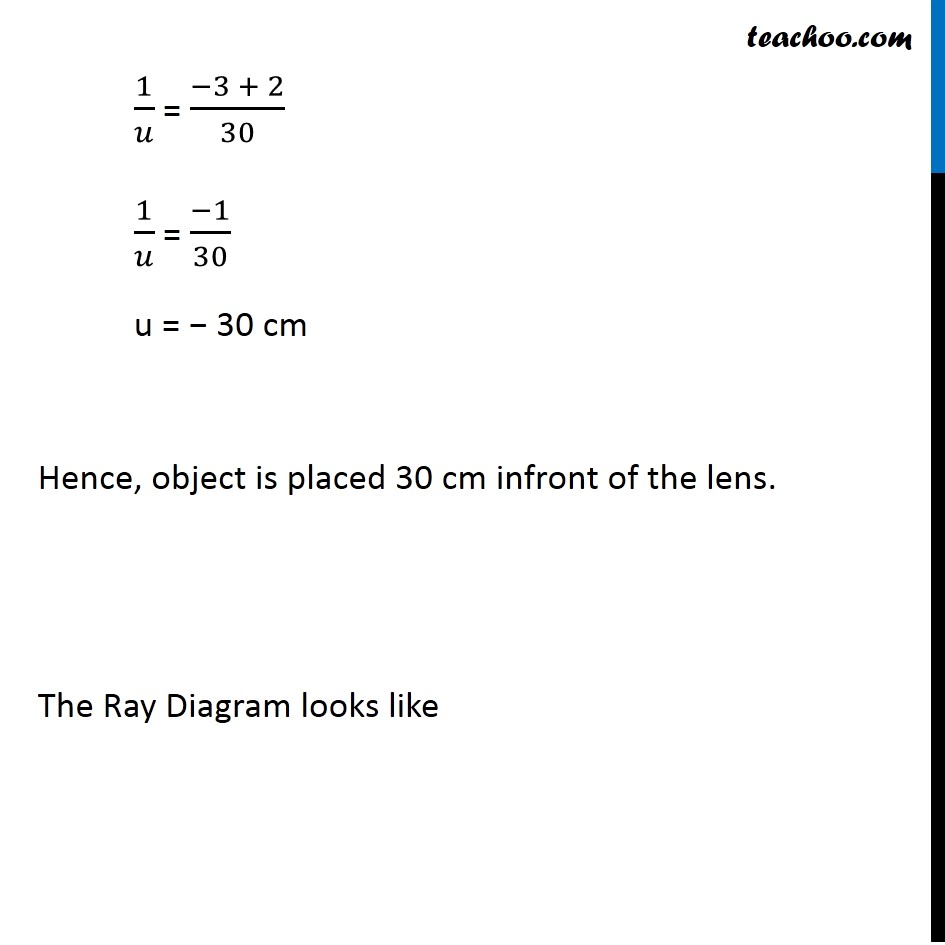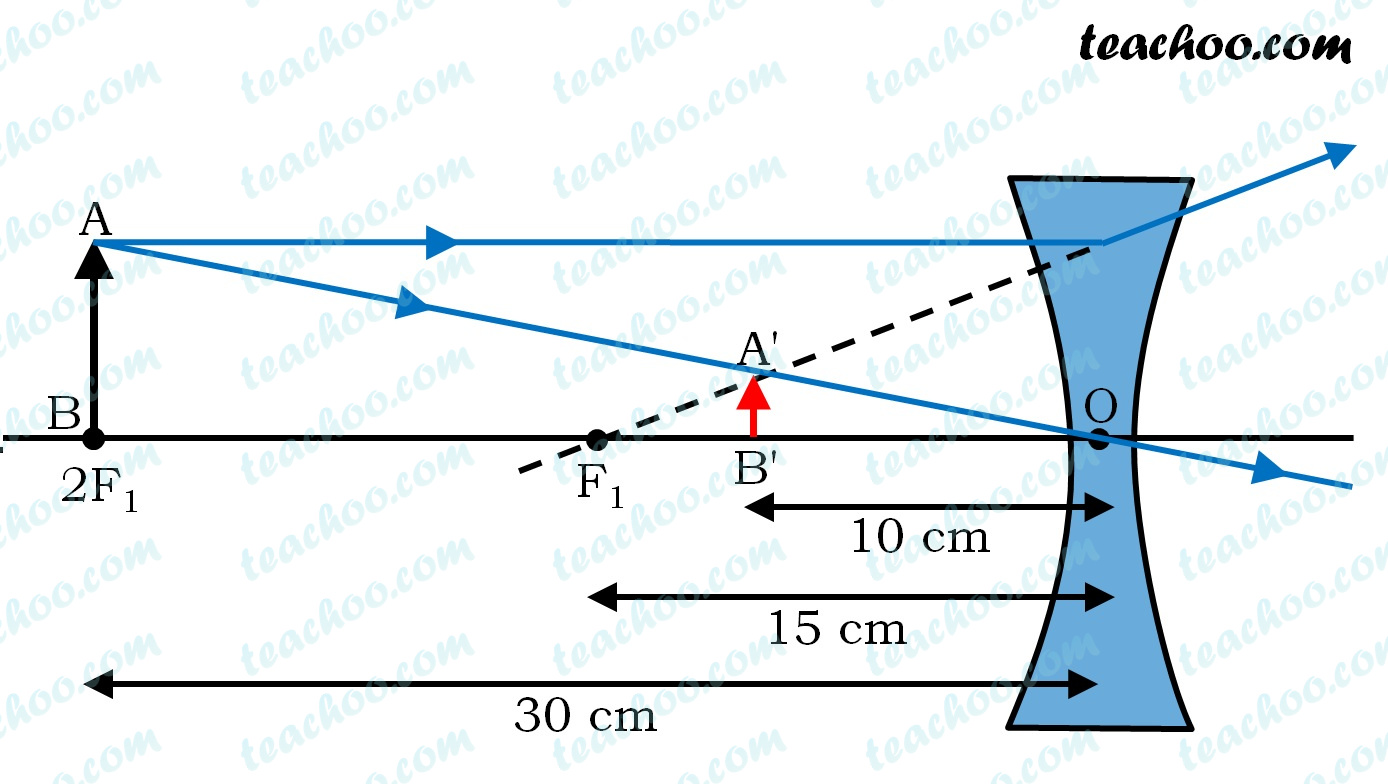NCERT Questions

Class 10
Chapter 10 Class 10 - Light - Reflection and Refraction

## A concave lens of focal length 15 cm forms an image 10 cm from the lens. How far is the object placed from the lens? Draw the ray diagram.Learn in your speed, with individual attention - Teachoo Maths 1-on-1 Class

### Transcript

NCERT Question 11 A concave lens of focal length 15 cm forms an image 10 cm from the lens. How far is the object placed from the lens? Draw the ray diagram Since the focal length of a concave lens is negative ∴ Focal length = f = −15 cm Also, A concave lens forms a virtual, erect image Hence, image distance will be negative Image distance = v = −10 cm Let the object distance = u Using lens formula, 1/𝑓 = 1/𝑣 − 1/𝑢 1/𝑓 − 1/𝑣 = (−1)/𝑢 (−1)/𝑢 = 1/𝑓 − 1/𝑣 1/𝑢 = 1/𝑣 − 1/𝑓 1/𝑢 = (−1)/10 − 1/((−15)) 1/𝑢 = (−1)/10 + 1/15 1/𝑢 = (−3 + 2)/30 1/𝑢 = (−1)/30 u = − 30 cm 1/𝑢 = (−3 + 2)/30 1/𝑢 = (−1)/30 u = − 30 cm Hence, object is placed 30 cm infront of the lens. The Ray Diagram looks like